# 数据结构与算法系列之数组## 什么是数组？

﻿

﻿

﻿

﻿

1. 数组是一种线性数据结构

2. 数组中存储的是连续的内存空间和相同类型的数据

﻿

#### 什么是线性数据结构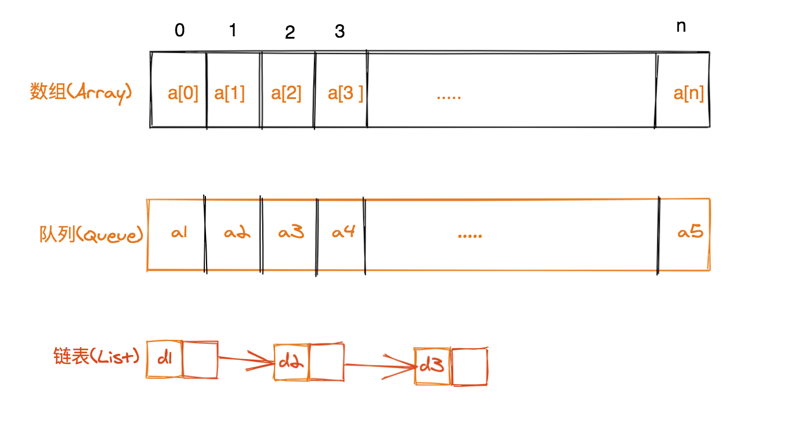﻿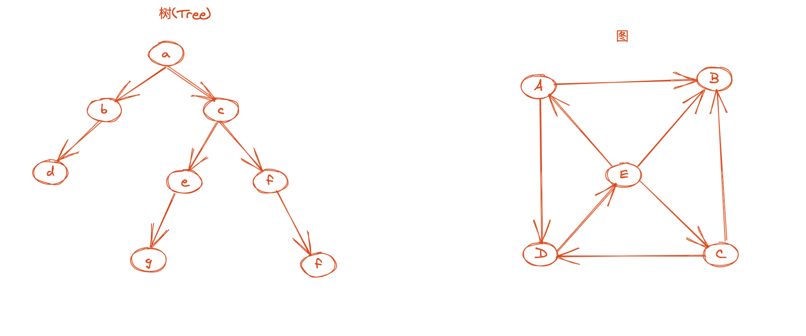﻿

#### 连续的内存空间和相同类型的数据

﻿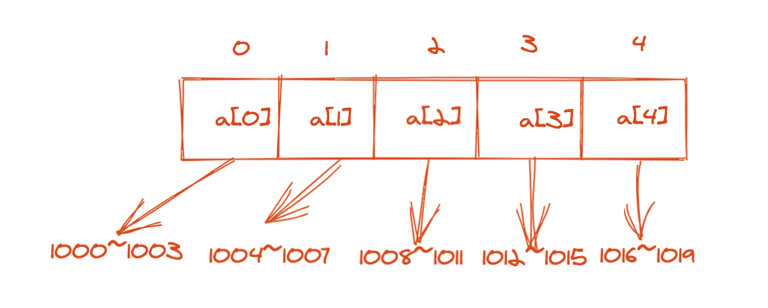﻿

a[i]_address = base_address + i * data_type_sizedata_type_size：表示数组中每个元素的大小。比如int类型，它占用的是4个字节，所以这里data_type_size就是4

﻿

﻿

## 数组相关操作

﻿

#### 插入元素

﻿

﻿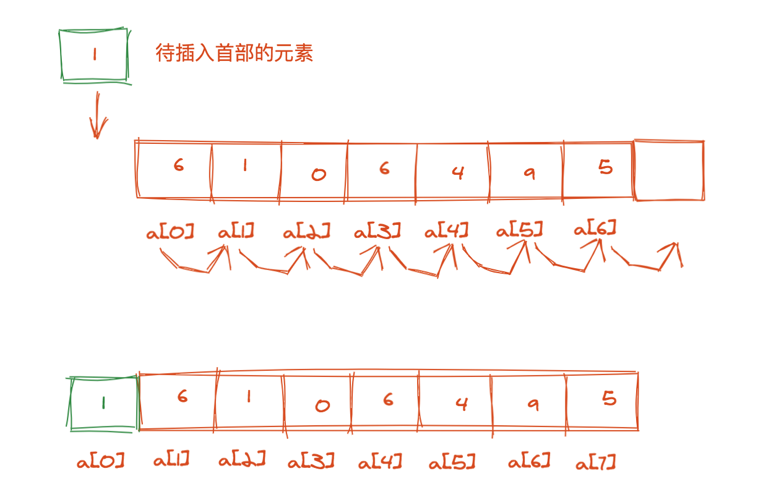﻿

function insertIntoHead($elem,$arr)    {        echo "原始数组：".PHP_EOL;        print_r($arr);$i = 0;        $len = count($arr);        while ($i <$len) {            $arr[$len-$i] =$arr[$len-$i-1];            $i++; }$arr = $elem; echo "头部插入元素之后结果：".PHP_EOL; print_r($arr);    }

﻿

﻿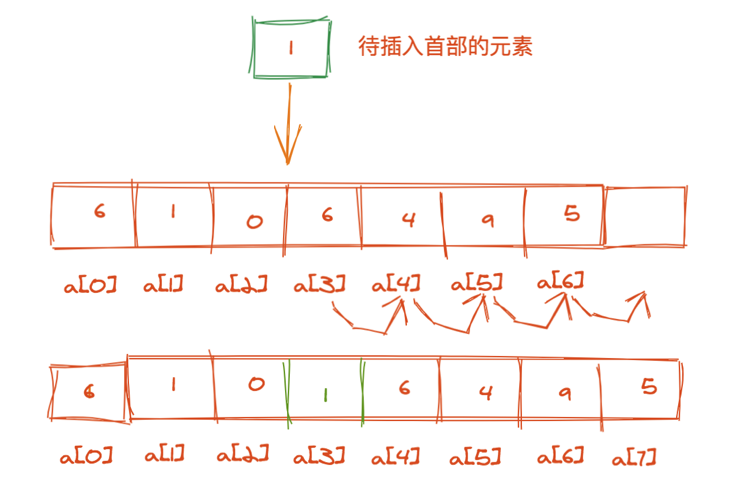﻿

function insertIntoMid($elem,$position, $arr) { echo "原始数组：".PHP_EOL; print_r($arr);        $len = count($arr);        $i =$position-1;        $value =$arr[$i];$arr[$i] =$elem;        $head =$i+1;        $offset =$position-1;        while ($i <$len) {            $arr[$len-$i+$offset] = $arr[$len-$i-1+$offset];            $i++; }$arr[$head] =$value;        echo "头部插入元素之后结果：".PHP_EOL;        print_r($arr); } ﻿ 数组尾部插入元素 ﻿ 在尾部插入元素，不需要移动元素，直接放在当前的末尾元素后边即可 ﻿ #### 删除元素 数组头部删除元素 ﻿ 删除数组头部元素，将所有的元素向前移动一位即可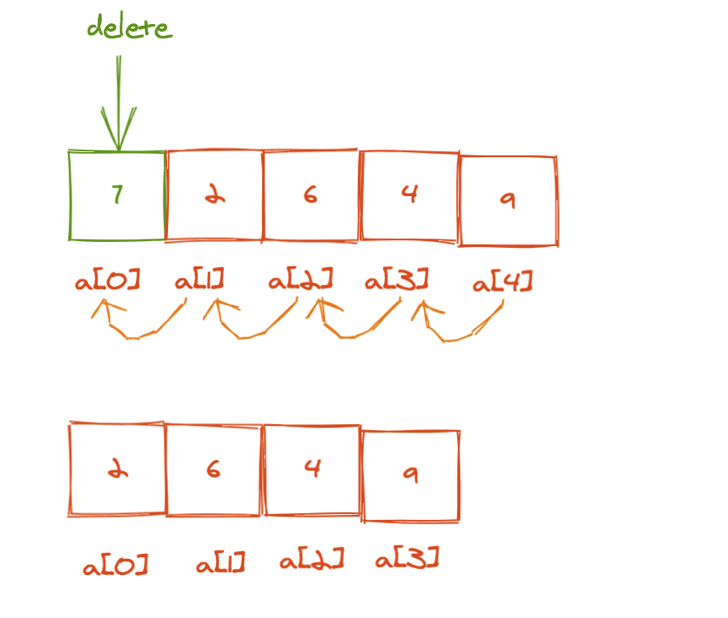﻿ 代码实现： function delHead($arr){        echo "删除前：".PHP_EOL;        print_r($arr); for ($i=0; $i<count($arr)-1; $i++) {$arr[$i] =$arr[$i+1]; } unset($arr[count($arr)-1]); echo "删除后".PHP_EOL; print_r($arr);}

﻿

﻿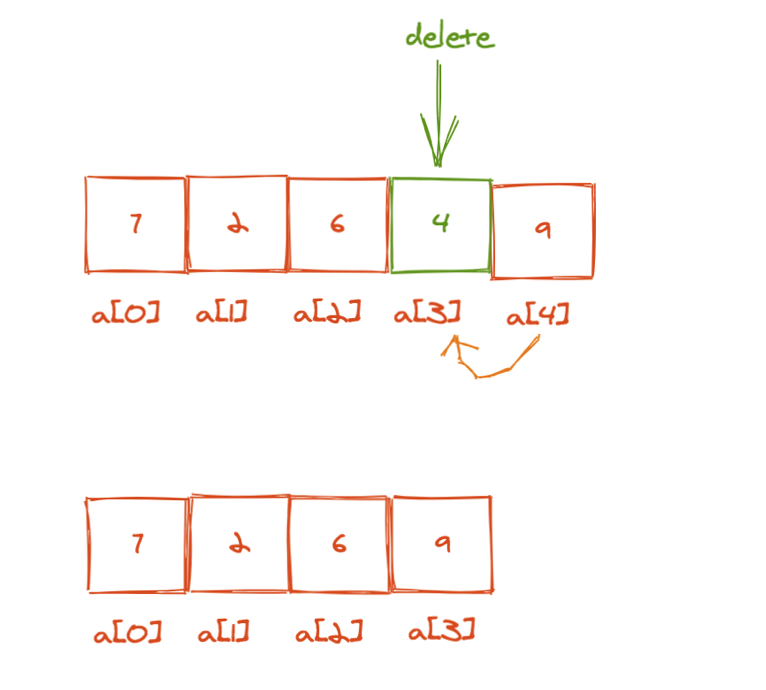﻿

function delMid($position,$arr){        echo "删除前：".PHP_EOL;        print_r($arr);$len = count($arr); for ($i=$position-1;$i<$len-1;$i++)   {            $arr[$i] = $arr[$i+1];        }        unset($arr[$len-1]);                echo "删除后".PHP_EOL;        print_r(\$arr);}

﻿

﻿

﻿

﻿

## 为什么大多数编程语言中，数组要从0开始编号，而不是从1开始呢？

a[k]_address = base_address + k * type_size

﻿

a[k]_address = base_address + (k-1)*type_size

﻿

﻿

《数据结构与算法之美》

《零基础学数据结构》

﻿﻿## 评论 (2 条评论)2020 年 09 月 14 日 12:15

2020 年 09 月 14 日 13:44